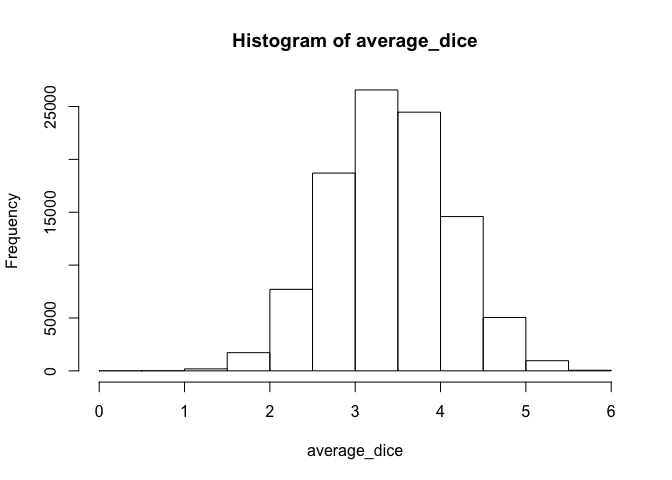# STAT 385 Lab 01

## What You Need For This Lab

• R and RStudio working on your personal computer.
• Read and understand the following lecture notes:

## Exercise 1: Rolling Dice

• GOAL: an R function named roll_dice() that simulates rolling 6 dice and compute the average outcome of the dice.
• Input: no input
• Output: a number presenting the average of the 6 dice outcomes.
roll_dice()
##  3.666667
roll_dice()
##  2.833333

When you finish writing your function, copy the following code segment and run it in your RStudio console:

average_dice <- replicate(n = 100000, expr = roll_dice())
hist(x = average_dice, breaks = seq(from = 0, to = 6, by = 0.5))## Exercise 2: Rolling Dice AGAIN

• I know this is so boring, but we will get to the exciting stuff soon!
• GOAL:
• An R function that rolls a pair of dices.
• One die is red and one die is green.
• These dices are configurable (meaning there might be more or fewer than 6 faces).
• Print out whether the outcome on the red die is greater than or equal to the out come on the green die.
• Function should have:
• Input:
• red: value of the faces of the red die. If no value is provided, the default value is 1:6.
• green: value of the faces of the green die. If no value is provided, the default value is 1:6.
• Output:
• TRUE if the outcome on the red die is greater than or equal to the out come on the green die.
• FALSE otherwise.

## Exercise 3: List of Lists

Here is the code to create the list used in this exercise:

instructor <- list("Ha", 24)
students <- list(list("Alex", 20), list("Dave", 21))
stat385 <- list(instructor, students)
stat385
## []
## [][]
##  "Ha"
##
## [][]
##  24
##
##
## []
## [][]
## [][][]
##  "Alex"
##
## [][][]
##  20
##
##
## [][]
## [][][]
##  "Dave"
##
## [][][]
##  21

Copy the above code and run it in your RStudio console.

Now, here are the tasks:

• Get Alex’s age.
• Get a list containing ONLY Dave’s name and age.
• Get Ha’s name.

## Exercise 4: Matrix

Here is the code to create the matrix used in this exercise:

m <- matrix(data = 1:16, nrow = 4, byrow = TRUE)
m
##      [,1] [,2] [,3] [,4]
## [1,]    1    2    3    4
## [2,]    5    6    7    8
## [3,]    9   10   11   12
## [4,]   13   14   15   16

Copy the above code and run it in your RStudio console.

Now, here are the tasks:

• Get the element on the 1st row, 3rd column.
• Get the element on the 3rd row, 2nd column.
• Get all the elements from the 1st row.
• Get all the elements from the 3rd column.
• Compute the sum of all the elements from the 1st row.
• Compute the average of all the elements from the 3rd column.